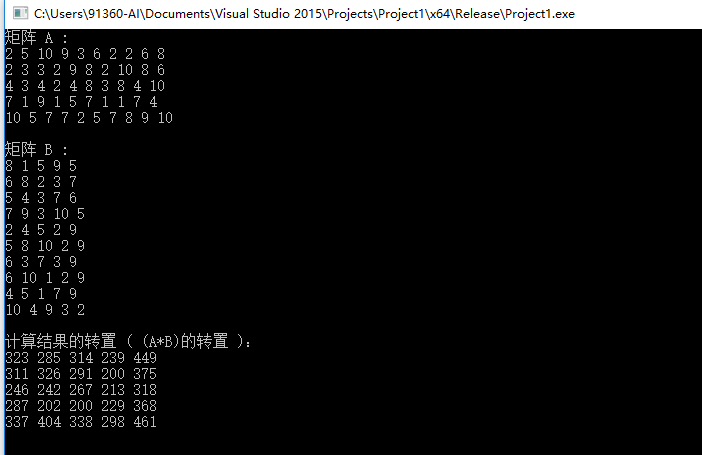# window10下CUDA9安装和vs2015配置

## 1.VS2013 + CUDA7.5 安装和配置

### 1.1 CUDA 7.5 安装

• 1.cuda官方下载网址，目前最新版本为cuda7.5，选择自己系统对应的版本下载安装程序。
• 2.运行cuda_ 7.5.18_windows.exe，程序会检测系统的兼容性如下图：• 3.选择“同意并继续”，进入下图安装过程 （备注：安装cuda时，要关闭所有的vs程序）• 4.等待大概半个小时后，安装程序终于完成了。

### 1.2 添加环境变量

CUDA_PATH = C:\Program Files\NVIDIA GPU Computing Toolkit\CUDA\v7.5
CUDA_PATH_V7_5 = C:\Program Files\NVIDIA GPU Computing Toolkit\CUDA\v7.5
CUDA_SDK_PATH = C:\ProgramData\NVIDIA Corporation\CUDA Samples\v7.5
CUDA_LIB_PATH = %CUDA_PATH%\lib\x64
CUDA_BIN_PATH = %CUDA_PATH%\bin
CUDA_SDK_BIN_PATH = %CUDA_SDK_PATH%\bin\win64
CUDA_SDK_LIB_PATH = %CUDA_SDK_PATH%\common\lib\x64

• 1
• 2
• 3
• 4
• 5
• 6
• 7
• 8

### 1.4 VS2013 + CUDA7.5配置

• 1.打开vs2013并创建一个空win32程序,创建一个cuda_samples的解决方案和cuda_test1项目：• 2.右键源文件–>添加–>新建项，如下图所示：• 3.选择NIVIDIA CUDA7.5中 CUDA C/C++file，并在名称那填上cuda_main• 4.选择cuda_test1，点击右键–>项目依赖项–>自定义生成• 5.选择CUDA7.5• 6.点击cuda_main.cu的属性1. 在配置属性–>常规–>项类型–>选择“CUDA C/C++”### 1.5 项目配置

#### 1.5.1.1 包含目录配置

• 1.右键点击项目属性–>属性–>配置属性–>VC++目录–>包含目录
• 2.添加包含目录：

#### 1.5.1.3 依赖项

1. 配置属性–>连接器–>输入–>附加依赖项
1. 添加库文件：
cublas.lib
cuda.lib
cudart.lib
cudart_static.lib
nvcuvid.lib
OpenCL.lib

### 1.5.2 x86(win32)

#### 1.5.2.1 包含目录配置

1. 右键点击项目属性–>属性–>配置属性–>VC++目录–>包含目录
1. 添加包含目录：

#### 1.5.2.3 依赖项

1. 配置属性–>连接器–>输入–>附加依赖项
1. 添加库文件：
cuda.lib
cudart.lib
cudart_static.lib
nvcuvid.lib
OpenCL.lib

### 1.6 样例

// CUDA runtime 库 + CUBLAS 库
#include "cuda_runtime.h"
#include "cublas_v2.h"
#include <time.h>
#include <iostream>

using namespace std;
// 定义测试矩阵的维度
int const M = 5;
int const N = 10;
int main()
{
// 定义状态变量
cublasStatus_t status;
// 在内存中为将要计算的矩阵开辟空间
float *h_A = (float*)malloc(N*M*sizeof(float));
float *h_B = (float*)malloc(N*M*sizeof(float));
// 在 内存 中为将要存放运算结果的矩阵开辟空间
float *h_C = (float*)malloc(M*M*sizeof(float));
// 为待运算矩阵的元素赋予 0-10 范围内的随机数
for (int i = 0; i<N*M; i++) {
h_A[i] = (float)(rand() % 10 + 1);
h_B[i] = (float)(rand() % 10 + 1);
}
// 打印待测试的矩阵
cout << "矩阵 A :" << endl;
for (int i = 0; i<N*M; i++){
cout << h_A[i] << " ";
if ((i + 1) % N == 0) cout << endl;
}
cout << endl;
cout << "矩阵 B :" << endl;
for (int i = 0; i<N*M; i++){
cout << h_B[i] << " ";
if ((i + 1) % M == 0) cout << endl;
}
cout << endl;
/*
** GPU 计算矩阵相乘
*/
// 创建并初始化 CUBLAS 库对象
cublasHandle_t handle;
status = cublasCreate(&handle);
if (status != CUBLAS_STATUS_SUCCESS)
{
if (status == CUBLAS_STATUS_NOT_INITIALIZED) {
cout << "CUBLAS 对象实例化出错" << endl;
}
getchar();
return EXIT_FAILURE;
}
float *d_A, *d_B, *d_C;
// 在 显存 中为将要计算的矩阵开辟空间
cudaMalloc(
(void**)&d_A, // 指向开辟的空间的指针
N*M * sizeof(float) //　需要开辟空间的字节数
);
cudaMalloc(
(void**)&d_B,
N*M * sizeof(float)
);
// 在 显存 中为将要存放运算结果的矩阵开辟空间
cudaMalloc(
(void**)&d_C,
M*M * sizeof(float)
);
// 将矩阵数据传递进 显存 中已经开辟好了的空间
cublasSetVector(
N*M, // 要存入显存的元素个数
sizeof(float), // 每个元素大小
h_A, // 主机端起始地址
1, // 连续元素之间的存储间隔
d_A, // GPU 端起始地址
1 // 连续元素之间的存储间隔
);
cublasSetVector(
N*M,
sizeof(float),
h_B,
1,
d_B,
1
);
// 同步函数
// 传递进矩阵相乘函数中的参数，具体含义请参考函数手册。
float a = 1; float b = 0;
// 矩阵相乘。该函数必然将数组解析成列优先数组
cublasSgemm(
handle, // blas 库对象
CUBLAS_OP_T, // 矩阵 A 属性参数
CUBLAS_OP_T, // 矩阵 B 属性参数
M, // A, C 的行数
M, // B, C 的列数
N, // A 的列数和 B 的行数
&a, // 运算式的 α 值
d_A, // A 在显存中的地址
N, // lda
d_B, // B 在显存中的地址
M, // ldb
&b, // 运算式的 β 值
d_C, // C 在显存中的地址(结果矩阵)
M //
);
// 同步函数
// 从 显存 中取出运算结果至 内存中去
cublasGetVector(M*M, // 要取出元素的个数
sizeof(float), // 每个元素大小
d_C, // GPU 端起始地址
1, // 连续元素之间的存储间隔
h_C, // 主机端起始地址
1 // 连续元素之间的存储间隔
);
// 打印运算结果
cout << "计算结果的转置 ( (A*B)的转置 )：" << endl;
for (int i = 0; i<M*M; i++){
cout << h_C[i] << " ";
if ((i + 1) % M == 0) cout << endl;
}
// 清理掉使用过的内存
free(h_A);
free(h_B);
free(h_C);
cudaFree(d_A);
cudaFree(d_B);
cudaFree(d_C);
// 释放 CUBLAS 库对象
cublasDestroy(handle);
getchar();
return 0;
}©️2019 CSDN 皮肤主题: 技术黑板 设计师: CSDN官方博客